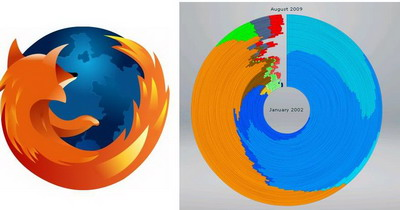Python程序员的进化

## Python程序员的进化

#### 新手程序员

```def factorial(x):
if x == 0:
return 1
else:
return x * factorial(x - 1)
print factorial(6)```

#### 第一年的刚学完Pascal的新手

```def factorial(x):
result = 1
i = 2
while i <= x:
result = result * i
i = i + 1
return result
print factorial(6)```

#### …(37 人打了分，平均分： 4.54 )Loading...

## 如何防范密码被破解(16 人打了分，平均分： 4.13 )Loading...

## 一个浏览器市场占有量的图

http://www.michaelvandaniker.com/labs/browserVisualization/(15 人打了分，平均分： 4.40 )Loading...

## 一个Windows 3.1的Web网站

http://www.michaelv.org/(8 人打了分，平均分： 4.25 )Loading...

## 各种流行的编程风格

#### …(46 人打了分，平均分： 4.89 )Loading...

## 最为奇怪的程序语言的特性

1、C语言中的数组

“Hello World”[i] 也可以写成 i[“Hello World”]

2、在Javascript中

‘5’ + 3 的结果是：’53’
‘5’ – 3 的结果是：2

3、C/C++中的Trigraphs

```int main() {
cout << "LOL??!";
}```(23 人打了分，平均分： 4.70 )Loading...
“第六感装置”的惊人潜力

## “第六感装置”的惊人潜力(26 人打了分，平均分： 4.58 )Loading...
PI小数点位数的新纪录

## PI小数点位数的新纪录

PI小数点后的位数据个数据的纪录被刷新了，被一台PC机刷新的。新的纪录把PI的小点数后面的位数整到了近2.7万亿位，太BT了。下面的链接是本次经录的通告：

http://bellard.org/pi/pi2700e9/announce.html

• PI后的小数点位数被计算到了2,699,999,990,000位。
• 为了保存计算结果，一共花了，1137GB的硬盘空间。
• 本次计算使用了价值2000欧元左右的PC机，CPU是Core i7 CPU at 2.93 GHz ，内存6GB，5个1.5TB的希捷硬盘。
• 操作系统使用的是Linux  64 bit Red Hat Fedora 10 distribution，7.5TB的硬盘被做成了RAID-0阵列，使用了ext4文件系统。
• 整个计算时间花了131天（4个半月），其中，103天用于计算PI的二进制结果，13天用于验证二进制结果，花了12天把二进制转成十进制，最后花了3天再验证了转换。
• 上一次的记录是2.577 万亿小数位，于2009年8月17日创造。其使用了超过百万欧元的超级计算机（Appro Xtreme-X3 Server）。(6 人打了分，平均分： 4.67 )Loading...
CPU的性价比

## CPU的性价比

http://paulisageek.com/compare/cpu/(9 人打了分，平均分： 4.89 )Loading...
2010 = 1+2-(3-4-5)*6*7*8-9

## 2010 = 1+2-(3-4-5)*6*7*8-9

这是一个数字游戏，使用123456789，并按照123456789的顺序，使用加减乘除以及括号，进行操作使其结果等于2010（原来的游戏是使其值为100，请看这里），那么会有多少种解法呢？下面是924种解法，其让我想起了“24点游戏”。

#### 使用单个数

2010 = 1+2-(3-4-5)*6*7*8-9
2010 = 1-(2+(3-4-5)*6*7)*8+9
2010 = 1+2+(3+4*(5+6*7+8))*9
2010 = 1+2*(3*4*(5+6)-7)*8+9
2010 = 1*2*3*(4*(5*6+7*8)-9)
2010 = 1+2+(3+4*(5-6+7*8))*9
2010 = (1-2-3+4*(5/6+7*8))*9
2010 = (1+2+3*4)*(5-6+(7+8)*9)
2010 = 1+2+((3*(4+5)+6)*7-8)*9
2010 = (1+2+3)*(4*(5*6+7*8)-9)
2010 = 1+2+3*(4*(5+6)*(7+8)+9)
2010 = (1*2/3)*((4+5)*6*7*8-9)
2010 = (1-2-3)*((4+5)/6-7*8*9)
2010 = (1*2+(3-4*(5/6-7))*8)*9
2010 = 1*(2+(3-4*(5/6-7))*8)*9
2010 = (1+2*(3+4))*(5-6+(7+8)*9)(14 人打了分，平均分： 4.29 )Loading...